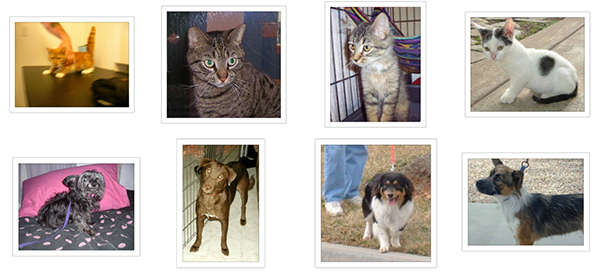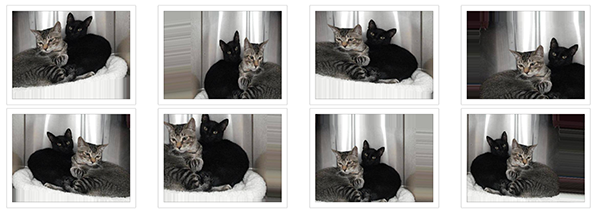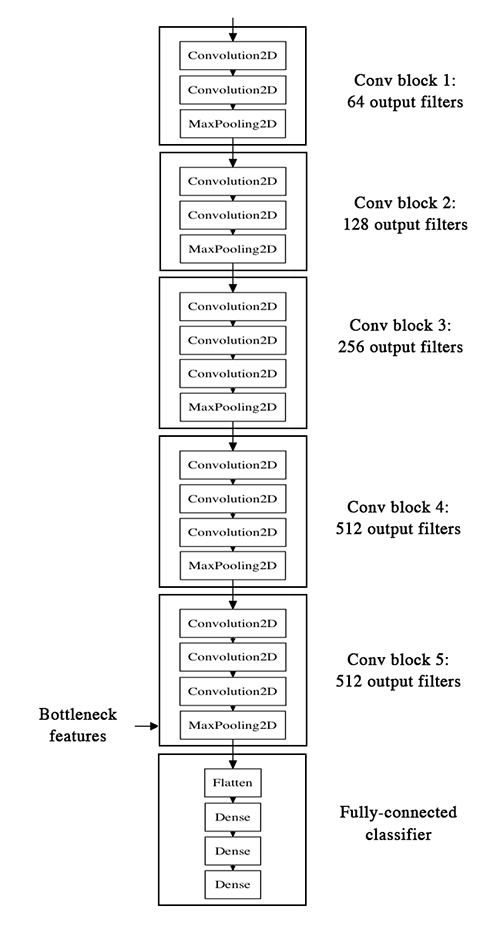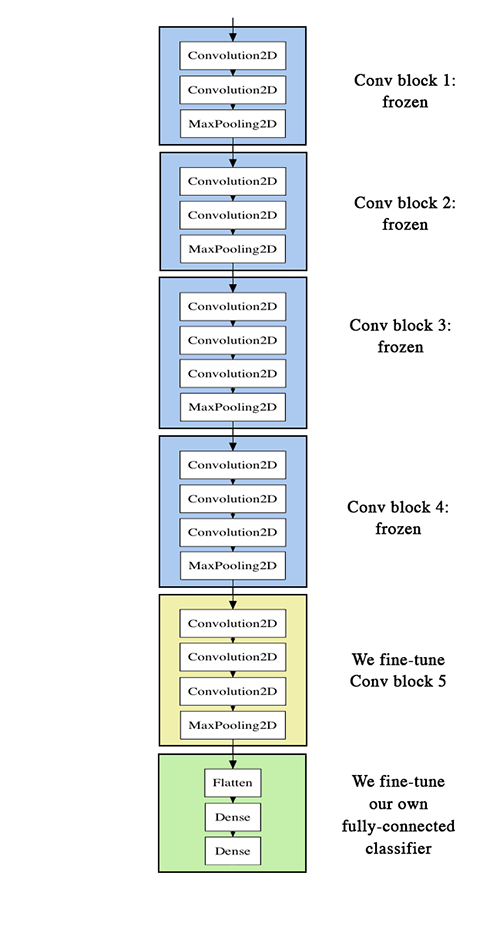# 【译文】使用很少的图片构建强大的图片分类模型

• 从头训练一个小的网络作为比较基准
• 使用预训练网络的bottleneck 特征
• 微调预训练网络的最后几层

• `fit_generator` 使用Python数据生成器来训练Keras模型
• `ImageDataGenerator` 实时的图像增强
• 层冻结和模型微调
• …其它

#### 我们的设定：只有2000个样本（每类1000个）

• 机器安装了Keras, SciPy, PIL。如果你有NVIDIA 显卡也可以用（需要安装cuDNN），不过由于我们处理的图片很少所以这不是必需的。
• 一个训练集目录和验证集目录，都包含以分类组织的子目录，这些子目录包含 .png或.jpg图片：
``````data/
train/
dogs/
dog001.jpg
dog002.jpg
...
cats/
cat001.jpg
cat002.jpg
...
validation/
dogs/
dog001.jpg
dog002.jpg
...
cats/
cat001.jpg
cat002.jpg
...
``````#### 数据预处理和数据增强

• 设定训练时图片的随机变换和标准化操作
• 通过 `.flow(data, labels)``flow_from_directory(directory)`来实例化批量增强图片的生成器。这些生成器随即可以作为`fit_generator`，``evaluate_generator` 和`predict_generator`等Keras 模型方法的输入。

``````from keras.preprocessing.image import ImageDataGenerator

datagen = ImageDataGenerator(
rotation_range=40,
width_shift_range=0.2,
height_shift_range=0.2,
rescale=1./255,
shear_range=0.2,
zoom_range=0.2,
horizontal_flip=True,
fill_mode='nearest')
``````

• `rotation_range` 是0~180的度数值，表示图片旋转的范围
• `width_shift``height_shift` 是宽度、长度的百分比，代表图片平移的范围
• `rescale` 表示在其它处理之前对图片的乘数。我们的原始图片是由0~255之间的RGB值构成的，这种值模型处理起来太大了（在典型的学习率下），所以需要通过缩放因子1/255把值缩放到0~1之间
• `shear_range` 表示随机应用错切
• `zoom_range` 表示图片内的随机缩放
• `horizontal_flip` 表示随机地水平翻转图片——当没有水平不对称的假设时(例如，真实世界的图片)。
• `fill_mode` 表示在旋转或水平/垂直平移后，对新像素的填充策略

``````from keras.preprocessing.image import ImageDataGenerator, array_to_img, img_to_array, load_img

datagen = ImageDataGenerator(
rotation_range=40,
width_shift_range=0.2,
height_shift_range=0.2,
shear_range=0.2,
zoom_range=0.2,
horizontal_flip=True,
fill_mode='nearest')

img = load_img('data/train/cats/cat.0.jpg')  #这是一个 PIL 图片
x = img_to_array(img)  # 这是一个 Numpy 数组，形状是 (3, 150, 150)
x = x.reshape((1,) + x.shape)  # 这是一个 Numpy 数组，形状是 (1, 3, 150, 150)

# 下面的 .flow() 命令生成一批随机变换的图片
# 然后保存到 `preview/` 目录
i = 0
for batch in datagen.flow(x, batch_size=1,
save_to_dir='preview', save_prefix='cat', save_format='jpeg'):
i += 1
if i > 20:
break  # 否则生成器会一直循环下去
``````#### 从头训练一个小卷积网络：40行代码达到80%准确率

``````from keras.models import Sequential
from keras.layers import Conv2D, MaxPooling2D
from keras.layers import Activation, Dropout, Flatten, Dense

model = Sequential()
model.add(Conv2D(32, (3, 3), input_shape=(3, 150, 150)))

#目前为止模型的输出维度 (height, width, features)
``````

``````model.add(Flatten())  # 3维数据特征转化为1维

model.compile(loss='binary_crossentropy',
optimizer='rmsprop',
metrics=['accuracy'])
``````

``````batch_size = 16

# 用于训练的图片增强配置
train_datagen = ImageDataGenerator(
rescale=1./255,
shear_range=0.2,
zoom_range=0.2,
horizontal_flip=True)

# 用于测试的图片增强配置:只设定rescale
test_datagen = ImageDataGenerator(rescale=1./255)

# 生成器从'data/train' 文件夹中读取数据, 可以无限生成增强的图片数据
train_generator = train_datagen.flow_from_directory(
'data/train',  # 目标文件夹
target_size=(150, 150),  # 所有图片尺寸重设为 150x150
batch_size=batch_size,
class_mode='binary')  # binary_crossentropy 损失函数需要二值标签

# 一个类似的生成器，用于验证集数据
validation_generator = test_datagen.flow_from_directory(
'data/validation',
target_size=(150, 150),
batch_size=batch_size,
class_mode='binary')
``````

``````model.fit_generator(
train_generator,
steps_per_epoch=2000 // batch_size,
epochs=50,
validation_data=validation_generator,
validation_steps=800 // batch_size)
model.save_weights('first_try.h5')  # 记得在训练后和训练中适时保存模型
``````

#### 使用预训练网络的bottleneck 特征：一分钟内达到90%准确率``````batch_size = 16

generator = datagen.flow_from_directory(
'data/train',
target_size=(150, 150),
batch_size=batch_size,
class_mode=None,  # 这个生成器不产生标签
shuffle=False)  # 保持数据顺序，先是1000只猫，然后是1000条狗
# 给定一个生成器yields批量的numpy数据，predict_generator会返回模型的输出
bottleneck_features_train = model.predict_generator(generator, 2000)
# 保存这些输出到 numpy数组
np.save(open('bottleneck_features_train.npy', 'w'), bottleneck_features_train)

generator = datagen.flow_from_directory(
'data/validation',
target_size=(150, 150),
batch_size=batch_size,
class_mode=None,
shuffle=False)
bottleneck_features_validation = model.predict_generator(generator, 800)
np.save(open('bottleneck_features_validation.npy', 'w'), bottleneck_features_validation)
``````

``````train_data = np.load(open('bottleneck_features_train.npy'))
# 由于特征是按猫狗的顺序产生的，所有构造标签很简单
train_labels = np.array( * 1000 +  * 1000)

validation_labels = np.array( * 400 +  * 400)

model = Sequential()

model.compile(optimizer='rmsprop',
loss='binary_crossentropy',
metrics=['accuracy'])

model.fit(train_data, train_labels,
epochs=50,
batch_size=batch_size,
validation_data=(validation_data, validation_labels))
model.save_weights('bottleneck_fc_model.h5')
``````

``````Train on 2000 samples, validate on 800 samples
Epoch 1/50
2000/2000 [==============================] - 1s - loss: 0.8932 - acc: 0.7345 - val_loss: 0.2664 - val_acc: 0.8862
Epoch 2/50
2000/2000 [==============================] - 1s - loss: 0.3556 - acc: 0.8460 - val_loss: 0.4704 - val_acc: 0.7725
...
Epoch 47/50
2000/2000 [==============================] - 1s - loss: 0.0063 - acc: 0.9990 - val_loss: 0.8230 - val_acc: 0.9125
Epoch 48/50
2000/2000 [==============================] - 1s - loss: 0.0144 - acc: 0.9960 - val_loss: 0.8204 - val_acc: 0.9075
Epoch 49/50
2000/2000 [==============================] - 1s - loss: 0.0102 - acc: 0.9960 - val_loss: 0.8334 - val_acc: 0.9038
Epoch 50/50
2000/2000 [==============================] - 1s - loss: 0.0040 - acc: 0.9985 - val_loss: 0.8556 - val_acc: 0.9075
``````

#### 微调预训练网络的最后几层

• 初始化VGG16 网络的卷积部分加载权重
• 在此基础上增加我们前面训练的全连接网络并加载权重
• 冻结最后的卷积模块之前的层• 为了微调模型，所有层都要从训练过的权重开始：比如你不能在预训练的卷积层上增加一个使用随机值初始化的全连接层。因为随机初始化的权重带来的大的更新会伤害卷积层的权重。所以在本例中我们先训练了一个全连接层的分类器，然后才将它和卷积层放在一起工作。
• 为了防止过拟合我们只微调最后的卷积层而不是整个网络，因为整个网络有非常大的熵容量因此很容易过拟合。底层的卷积网络学到的特征是更基础的特征，没有高层特征那么抽象，所以固定最开始几层的特征（更加基础的特征）只训练最后一层是合理的。
• 微调应该用很小的学习率，而且一般用SGD 优化器而不是那些自适应的优化器比如RMSProp。这是为了保持很小量级的权重更新，不伤害网络已经学到的特征。

``````# 在卷积层上增加分类器
top_model = Sequential()

# 注意为了微调训练，全连接分类器也应该训练过
# classifier, including the top classifier,
# in order to successfully do fine-tuning

# 添加到卷积层之上
``````

``````batch_size = 16

# 准备数据增强
train_datagen = ImageDataGenerator(
rescale=1./255,
shear_range=0.2,
zoom_range=0.2,
horizontal_flip=True)

test_datagen = ImageDataGenerator(rescale=1./255)

train_generator = train_datagen.flow_from_directory(
train_data_dir,
target_size=(img_height, img_width),
batch_size=batch_size,
class_mode='binary')

validation_generator = test_datagen.flow_from_directory(
validation_data_dir,
target_size=(img_height, img_width),
batch_size=batch_size,
class_mode='binary')

# 微调模型
model.fit_generator(
train_generator,
steps_per_epoch=nb_train_samples // batch_size,
epochs=epochs,
validation_data=validation_generator,
validation_steps=nb_validation_samples // batch_size)
``````

50轮次训练后，本方法可以到达0.94的准确率。很大的进步！

• 更激进的数据增强
• 更激进的dropout
• 使用L1 和L2 正则化（又称“权重衰减”）
• 多微调一层（同时用更大的正则化）